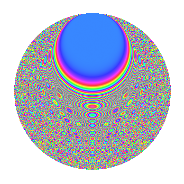# Properties

 Label 2015.2.doLevel 2015 Weight 2 Character orbit do Rep. character $$\chi_{2015}(161,\cdot)$$ Character field $$\Q(\zeta_{12})$$ Dimension 592 Sturm bound 448

# Related objects

## Defining parameters

 Level: $$N$$ = $$2015 = 5 \cdot 13 \cdot 31$$ Weight: $$k$$ = $$2$$ Character orbit: $$[\chi]$$ = 2015.do (of order $$12$$ and degree $$4$$) Character conductor: $$\operatorname{cond}(\chi)$$ = $$403$$ Character field: $$\Q(\zeta_{12})$$ Sturm bound: $$448$$

## Dimensions

The following table gives the dimensions of various subspaces of $$M_{2}(2015, [\chi])$$.

Total New Old
Modular forms 912 592 320
Cusp forms 880 592 288
Eisenstein series 32 0 32

## Trace form

 $$592q + 36q^{6} + 4q^{7} + 24q^{8} + 288q^{9} + O(q^{10})$$ $$592q + 36q^{6} + 4q^{7} + 24q^{8} + 288q^{9} + 12q^{11} - 24q^{14} - 544q^{16} + 44q^{18} - 4q^{19} + 8q^{20} + 48q^{21} - 24q^{22} - 72q^{24} + 24q^{26} - 52q^{31} + 88q^{32} + 32q^{33} + 12q^{34} + 12q^{37} - 8q^{39} + 4q^{41} + 132q^{44} - 8q^{45} - 96q^{52} - 72q^{53} + 156q^{57} - 24q^{59} - 24q^{63} + 272q^{66} - 8q^{67} + 72q^{68} + 16q^{70} + 8q^{71} - 136q^{72} - 36q^{73} - 120q^{74} - 56q^{76} - 32q^{78} - 144q^{79} + 16q^{80} - 312q^{81} - 12q^{83} + 144q^{84} + 120q^{86} - 16q^{87} + 240q^{93} + 32q^{94} - 384q^{96} + 8q^{97} + 20q^{98} + 120q^{99} + O(q^{100})$$

## Decomposition of $$S_{2}^{\mathrm{new}}(2015, [\chi])$$ into irreducible Hecke orbits

The newforms in this space have not yet been added to the LMFDB.

## Decomposition of $$S_{2}^{\mathrm{old}}(2015, [\chi])$$ into lower level spaces

$$S_{2}^{\mathrm{old}}(2015, [\chi]) \cong$$ $$S_{2}^{\mathrm{new}}(403, [\chi])$$$$^{\oplus 2}$$